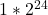Question

Bacteria multiply at an alarming rate when bacteria splits into two new cells, thus doubling. If we start with only one bacteria which can double every hour, how many bacteria will we have by the end of one day?

1.thuthao=16777216

Step-by-step explanation:

y=C*2^t is the equation for this type of growth

C is starting population and t is time

C=1 and t=24 (1 day= 24 hours)

Solve

2.quangkhai

2^24 = 16,777,216 bacterias.
Step-by-step explanation: